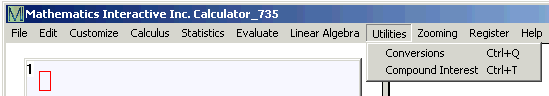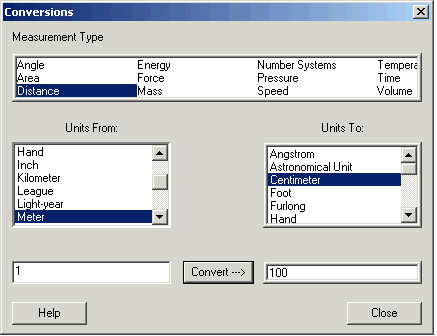# Distance Conversions

There are many different units used to measure length and distance.

You can convert a distance in one unit to another unit by clicking Utilities and then Conversions.Holding the Control key and typing q has the same result.

In the Conversions window select Distance.Click the unit you have in the Units From: box, and the units you want in the Units To: box.

Enter the number in the lower left box and click Convert.

Above shows that 1 meter is 100 centimeters.

The conversions are based on:

1 meter = 100 centimeters = 1000 millimeters

1 meter = 10 000 000 000 Angstroms

1 meter = 1 000 000 microns = 1 000 000 000 nanometers

1 kilometer = 1000 meters

1 Nautical mile = 1852 meters

1 league = 3 miles

1 mile = 5280 feet

1 foot = 12 inches

1 yard = 3 feet

1 fur = 660 feet

1 hand = 4 inches

1 inch = 2.54 centimeters

1 Astronomical unit = 149 597 870 kilometers

1 light-year = 63 241.0774 Astronomical units

1 parsec =  3.085 677 6 x 1016 meters